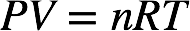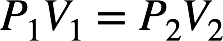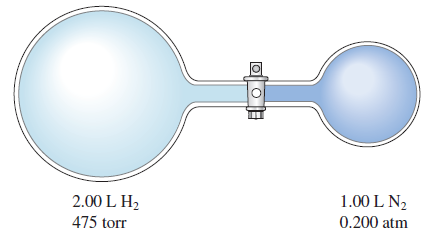# Problem: Consider the flasks in the following diagram. What are the final partial pressures of H 2 and N2 after the stopcock between the two flasks is opened? (Assume the final volume is 3.00 L.) What is the total pressure (in torr)?

###### FREE Expert Solution

Recall: The ideal gas law is as follows:We can see that the pressure and volume of a gas are related to the number of moles of gas, the universal gas constant, and the temperature of the gas.

For a given number of moles of gas at a constant temperature, the initial and final pressure and volume of the gas are related by Boyle's law.92% (169 ratings)###### Problem Details

Consider the flasks in the following diagram. What are the final partial pressures of H 2 and N2 after the stopcock between the two flasks is opened? (Assume the final volume is 3.00 L.) What is the total pressure (in torr)?Frequently Asked Questions

What scientific concept do you need to know in order to solve this problem?

Our tutors have indicated that to solve this problem you will need to apply the Partial Pressure concept. You can view video lessons to learn Partial Pressure. Or if you need more Partial Pressure practice, you can also practice Partial Pressure practice problems.

What professor is this problem relevant for?

Based on our data, we think this problem is relevant for Professor Brunold's class at UW-MADISON.

What textbook is this problem found in?

Our data indicates that this problem or a close variation was asked in Chemistry: An Atoms First Approach - Zumdahl Atoms 1st 2nd Edition. You can also practice Chemistry: An Atoms First Approach - Zumdahl Atoms 1st 2nd Edition practice problems.